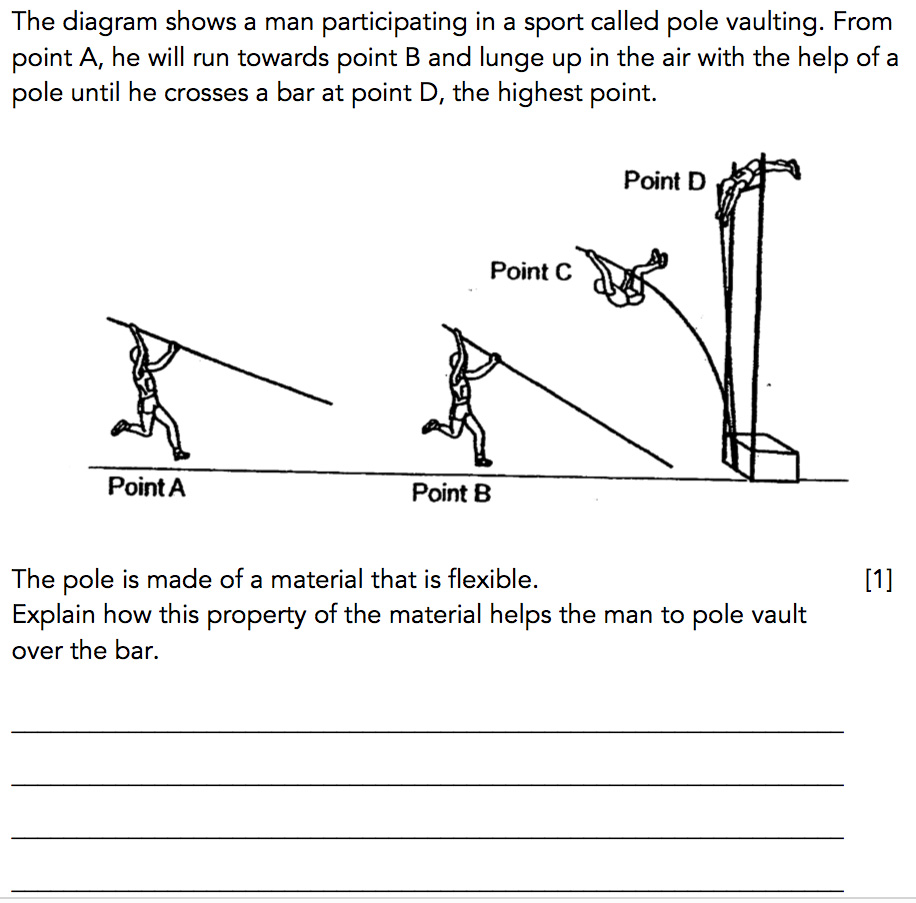In today’s article, I will be focusing on another type of potential energy – Elastic Potential Energy.

“Where can Elastic Potential Energy be commonly found?”

The answer that most students would give is, “Elastic Potential Energy can be found commonly in springs or rubber bands”.

However, there is a misconception in this answer. Before I elaborate on this, let me clarify some commonly asked questions.

“Does this mean that a rubber band or a spring that is lying on the table possess elastic potential energy?”

“Would a rubber band or a spring that is lying on the table move by itself?”

The answer to both of the above questions is “No”. A rubber band or a spring that is lying on the table does not possess elastic potential energy and is unable to move by itself unless a force is applied to it.

## An Accurate Answer To This Question

“Elastic potential energy can be commonly found in stretched or compressed springs and stretched rubber bands”.

The key words are “stretched” and “compressed”.

Only objects that are stretched or compressed possess elastic potential energy. The more stretched or compressed the object is, the greater the amount of elastic potential energy possessed by the object.

When the stretched or compressed object is released, the elastic potential energy that is possessed by the object would be converted to kinetic energy, causing the object to move forward.

Now that we’ve clarified this misconception, let us now apply the correct concepts to the question below.

## QuestionPHPS/2014/P6/SA2/Q43

In this question, the man is participating in a sport known as pole vaulting.

The man will carry a long pole at Point A and run towards Point B. At Point B, he will use the long pole to lunge up in the air, before crossing the bar at Point D. Before we answer the question accurately, let us analyse the type of energy possessed by the man at Point B and Point D.

Since the man ran towards Point B, the man possesses kinetic energy at Point B. At Point D, the man was hoisted in the air and cross over the bar placed at a height above the ground. Therefore, he possesses gravitational potential energy at Point D.

Watch this video to see pole vaulting in action:

## Thought Process

The question stated that the pole is made of a material that is flexible.

This means the pole is able to bend, as seen at Point C. When the pole is bent (stretched), it possesses elastic potential energy.

This elastic potential energy stored in the pole is converted to the kinetic energy of the man, allowing the man to move in the air.

This kinetic energy in the man is then converted to gravitational potential energy, thus causing him to move high up in the air to cross the bar.

With this understanding, we will be able to answer this question without much difficulty by incorporating the thought process above.

“When the pole bends, it stores elastic potential energy, which is converted to kinetic energy of the man, which is then converted to gravitational potential energy of the man. Therefore, this allows him to move upwards and over the bar.”If you like our methodology, we’ve some upcoming workshops: